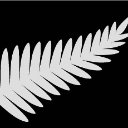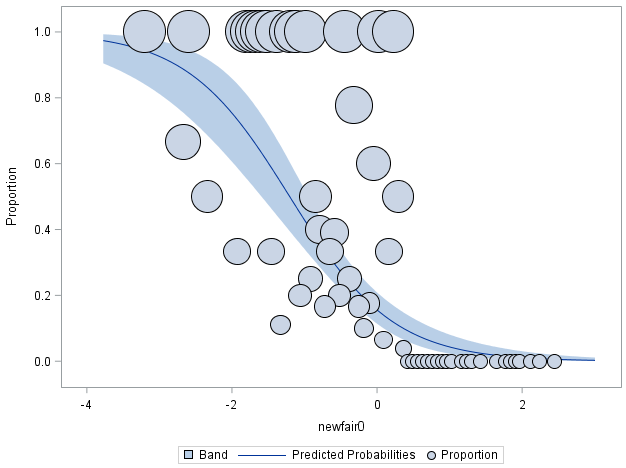## Proportionally Sized Bubble Plots

Hey there,

I have here an example of logistic regression, which spits out the graph of probabilty curve. On this graph, each dot represent all kids that scored a certain standard score (z score) on the NEWFAIR0 test. Now, I want the dots to reflect the numerus they represent, such that if a dot represents one kid, it should be smaller, and if it represents, say, 15 kids, it should be bigger. A dot in my data can represent from 1 to 31 kids. Here is my data and my code for logistic regression, but I dont know how to make the size of the dots proportional to the numerus of kids they represent. Thanks!!

data done4; input newfair0 parentdys N;

datalines;

-3.21 1 1

-2.67 2 3

-2.6 1 1

-2.34 2 4

-1.93 1 3

-1.8 1 1

-1.73 2 2

-1.66 2 2

-1.59 2 2

-1.53 1 1

-1.46 1 3

-1.39 1 1

-1.33 1 9

-1.19 2 2

-1.12 3 3

-1.06 1 5

-0.99 2 2

-0.92 1 4

-0.85 1 2

-0.79 2 5

-0.72 2 12

-0.65 1 3

-0.59 9 23

-0.52 1 5

-0.45 1 1

-0.38 1 4

-0.32 7 9

-0.25 1 6

-0.18 1 10

-0.11 3 17

-0.05 3 5

0.02 1 1

0.09 2 31

0.16 1 3

0.22 2 2

0.29 1 2

0.36 1 25

0.42 0 6

0.49 0 6

0.56 0 1

0.63 0 9

0.69 0 5

0.76 0 4

0.83 0 23

0.9 0 3

0.96 0 5

1.03 0 2

1.16 0 3

1.23 0 5

1.3 0 1

1.43 0 1

1.64 0 1

1.77 0 1

1.84 0 5

1.91 0 3

1.97 0 1

2.11 0 7

2.24 0 2

2.44 0 3

;

run;

ods graphics on;

proc logistic data=done4 plots(only)=(roc(id=obs) effect);

model parentdys/n=newfair0 / scale=none

clparm=wald

clodds=pl

rsquare;

units newfair0=1.00;

run;

ods graphics off;1 ACCEPTED SOLUTION

Accepted SolutionsChrisNZ
Tourmaline | Level 20

## Re: Proportionally Sized Bubble Plots

Something like this. You have to add the bubble size you want.

``````
ods graphics on;
ods output EffectPlot=EffectPlot;
proc logistic data=done4 plots(only)=(roc(id=obs) effect);
model parentdys/n=newfair0 / scale=none
clparm=wald
clodds=pl
rsquare;
units newfair0=1.00; output out=t/ profile;
run;

ods graphics off;

proc sgplot data= EffectPlot;
band   x=_XVAR     upper=_UPPER  lower=_LOWER ;
spline x=_XVAR     y=_PROB;
bubble x=_XVAR_OBS y=_PROPORTION size=_PROPORTION ;
run;``````6 REPLIES 6

## Re: Proportionally Sized Bubble Plots

Thank you. I have already looked at that website. Even though it is helpful in general, it does not help a lot in my case where the probabilities on the y axis are generated by the log regression, so you cannot define them as y=.... in the proc template. That's my main issue - what the code would be for y

## Re: Proportionally Sized Bubble Plots

There are times when to get the graph you want you generate a data set from a procedure and plot that with tools with more controls.

Most of the analysis procedure charts are designed more for analysis than publication.

## Re: Proportionally Sized Bubble Plots

Thanks. Any ideas how i could do that in my case?ChrisNZ
Tourmaline | Level 20

## Re: Proportionally Sized Bubble Plots

Something like this. You have to add the bubble size you want.

``````
ods graphics on;
ods output EffectPlot=EffectPlot;
proc logistic data=done4 plots(only)=(roc(id=obs) effect);
model parentdys/n=newfair0 / scale=none
clparm=wald
clodds=pl
rsquare;
units newfair0=1.00; output out=t/ profile;
run;

ods graphics off;

proc sgplot data= EffectPlot;
band   x=_XVAR     upper=_UPPER  lower=_LOWER ;
spline x=_XVAR     y=_PROB;
bubble x=_XVAR_OBS y=_PROPORTION size=_PROPORTION ;
run;``````## Re: Proportionally Sized Bubble Plots

Thanks!! That's exactly what I needed - a code that gets me started from somewhere. Will look into it in more detail.

Discussion stats
• 6 replies
• 726 views
• 0 likes
• 4 in conversation